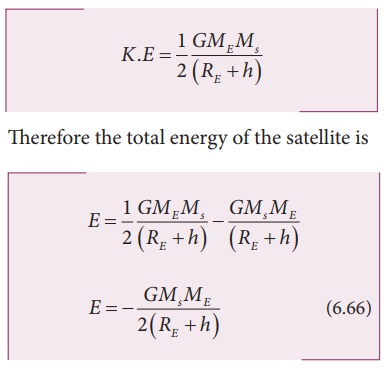Home | | Physics 11th std | Energy of an Orbiting Satellite

# Energy of an Orbiting Satellite

The total energy of the satellite is the sum of its kinetic energy and the gravitational potential energy.

Energy of an Orbiting Satellite

The total energy of a satellite orbiting the Earth at a distance h from the surface of Earth is calculated as follows; The total energy of the satellite is the sum of its kinetic energy and the gravitational potential energy. The potential energy of the satellite is,Here Ms - mass of the satellite, ME - mass of the Earth, RE - radius of the Earth. The Kinetic energy of the satellite isHere v is the orbital speed of the satellite and is equal toSubstituting the value of v in (6.64), the kinetic energy of the satellite becomes,The negative sign in the total energy implies that the satellite is bound to the Earth and it cannot escape from the Earth.

As h approaches Ōł×, the total energy tends to zero. Its physical meaning is that the satellite is completely free from the influence of EarthŌĆÖs gravity and is not bound to Earth at large distances.

## EXAMPLE 6.10

Calculate the energy of the (i) Moon orbiting the Earth and (ii) Earth orbiting the Sun.

## Solution

Assuming the orbit of the Moon to be circular, the energy of Moon is given by,where  ME is the mass  of Earth 6.02 ├Ś1024  kg; Mm is the mass of Moon 7.35 ├Ś1022 kg; and R  is the distance between the Moon and the center of the Earth 3.84 ├Ś105 kmThe negative energy implies that the Moon is bound to the Earth.

Same method can be used to prove that the energy of the Earth is also negative.

Study Material, Lecturing Notes, Assignment, Reference, Wiki description explanation, brief detail
11th Physics : UNIT 6 : Gravitation : Energy of an Orbiting Satellite |